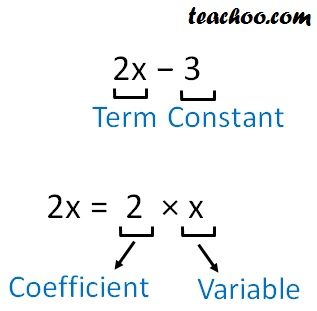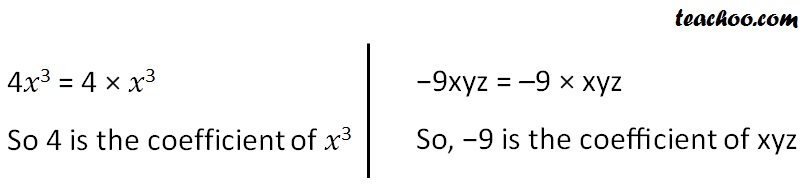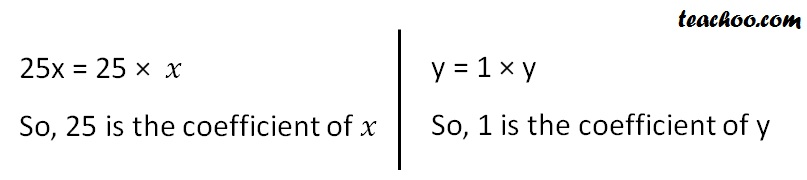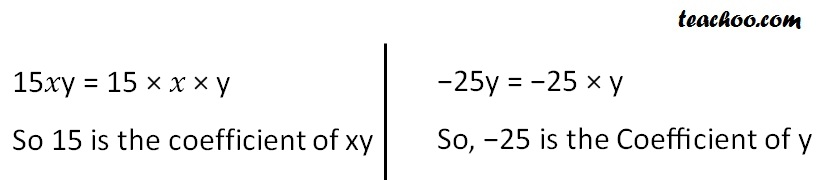Terms, Factors and Coefficients

Chapter 12 Class 7 Algebraic Expressions
Concept wise

Suppose our expression is

2𝑥 − 3

Here we consider terms other than constantNumber multiplied to the variable is the coefficient

Here, 2 is the coefficient of 2x

### For 4x 3 − 9xyz### For 25x + y### For 15𝑥y − 25y + 18Get live Maths 1-on-1 Classs - Class 6 to 12

### Transcript

For 4x3 − 9xyz 4𝑥3 = 4 × 𝑥3 So 4 is the coefficient of 𝑥3 −9xyz = –9 × xyz So, −9 is the coefficient of xyz For 25x + y 25x = 25 × 𝑥 So, 25 is the coefficient of 𝑥 y = 1 × y So, 1 is the coefficient of y For 15𝑥y − 25y + 18 15𝑥y = 15 × 𝑥 × y So 15 is the coefficient of xy −25y = −25 × y So, −25 is the Coefficient of y